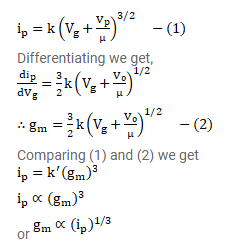# The plate current in a triode can be written as

Question:

The plate current in a triode can be written as $\mathrm{i}_{\mathrm{p}}=\mathrm{k}\left(\mathrm{V}_{\mathrm{g}}+\frac{\mathrm{V}_{\mathrm{p}}}{\mu}\right)^{3 / 2}$

Show that the mutual conductance is proportional to the cube root of the plate current.

Solution: